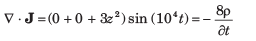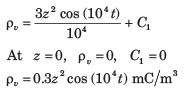Courses

# Maxwell’S Equations - MCQ Test

## 20 Questions MCQ Test GATE ECE (Electronics) 2022 Mock Test Series | Maxwell’S Equations - MCQ Test

Description
This mock test of Maxwell’S Equations - MCQ Test for Railways helps you for every Railways entrance exam. This contains 20 Multiple Choice Questions for Railways Maxwell’S Equations - MCQ Test (mcq) to study with solutions a complete question bank. The solved questions answers in this Maxwell’S Equations - MCQ Test quiz give you a good mix of easy questions and tough questions. Railways students definitely take this Maxwell’S Equations - MCQ Test exercise for a better result in the exam. You can find other Maxwell’S Equations - MCQ Test extra questions, long questions & short questions for Railways on EduRev as well by searching above.
QUESTION: 1

### A perfectly conducting filament containing a 250Ω​ resistor is formed into a square as shown in fig.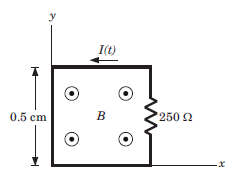​ ​ ​   Que: If B = 6 cos (120πt - 300 )uz T, then the value of I(t) is

Solution: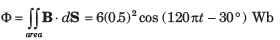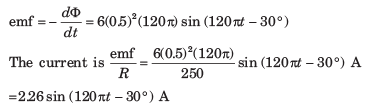QUESTION: 2

### A perfectly conducting filament containing a 250Ω​ resistor is formed into a square as shown in fig.Que: If B = 2 cosπ(ct - y) uz  μT, where c is the velocity of light, then I(t) is

Solution: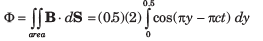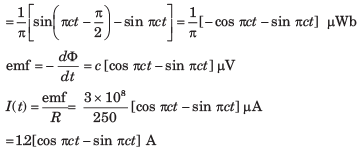QUESTION: 3

### Consider the fig. The rails have a resistance of 2 Ω/m. The bar moves to the right at a constant speed of 9 m/s in a uniform magnetic field of 0.8 T. The bar is at x = 2 m at t = 0.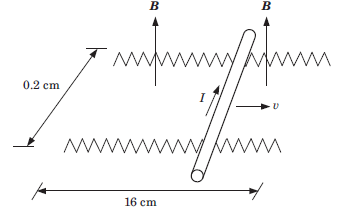Que: If 6 Ω resistor is present across the left-end with the right end open-circuited, then at t = 0.5 sec the current I is

Solution:

The flux in the left-hand closed loop is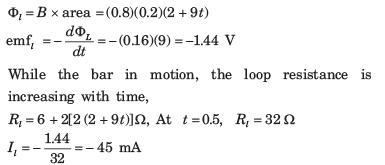QUESTION: 4

Consider the fig. The rails have a resistance of 2 Ω/m. The bar moves to the right at a constant speed of 9 m/s in a uniform magnetic field of 0.8 T. The bar is at x = 2 m at t = 0.Que: If 6 Ω resistor is present across each end, then I at 0.5 sec is

Solution:

In this case, there will be contribution to the current from the right loop, which is now closed. The flux in the right loop, whose area decreases with time, is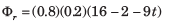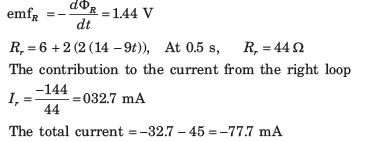QUESTION: 5

The internal dimension of a coaxial capacitor is a = 1.2 cm, b = 4 cm and c = 40 cm. The homogeneous material inside the capacitor has the parameter ε = 10-11 F/m, μ = 10-5H/m and  σ =10-S/m.The electric field intensity is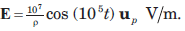Que: The current density J is

Solution: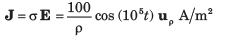QUESTION: 6

The internal dimension of a coaxial capacitor is a = 1.2 cm, b = 4 cm and c = 40 cm. The homogeneous material inside the capacitor has the parameter ε = 10-11 F/m, μ = 10-5H/m and  σ =10-S/m.The electric field intensity isQue: The quality factor of the capacitor is

Solution:

Total conduction current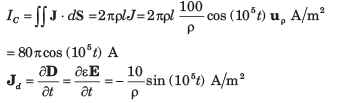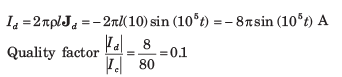QUESTION: 7

The following fields exist in charge free regions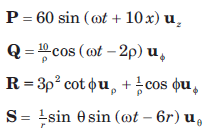The possible electromagnetic fields are

Solution: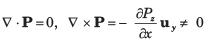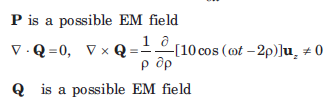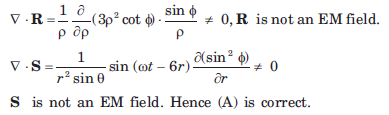QUESTION: 8

A parallel-plate capacitor with plate area of 5 cmand plate separation of 3 mm has a voltage 50 sin (103 t) V applied to its plates. If εr = 2, the displacement current is

Solution: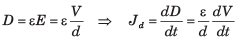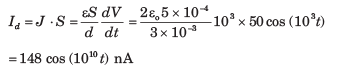QUESTION: 9

In a coaxial transmission line (εr = 1), the electric field intensity is given by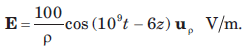The displacement current density is

Solution: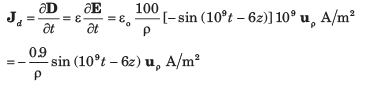QUESTION: 10

Consider the region defined by |x|,|y| and |z| < 1. Let ε = 5ε0 , μ = 4μo  and σ = 0 the displacement current densityJd  = 20cos(1.5 x 108 t - ax)uy μA/m2. Assume no DC fields are present.

Solution: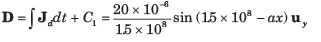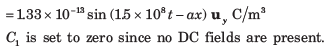QUESTION: 11

Consider the region defined by |x|,|y| and |z| < 1. Let ε = 5ε0 , μ = 4μo  and σ = 0 the displacement current densityJd  = 20cos(1.5 x 108 t - ax)uy μA/m2. Assume no DC fields are present.

Que: The magnetic field intensity is

Solution: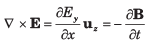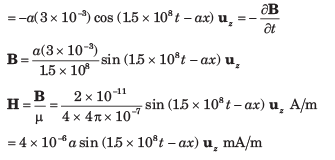QUESTION: 12

Consider the region defined by |x|,|y| and |z| < 1. Let ε = 5ε0 , μ = 4μo  and σ = 0 the displacement current densityJd  = 20cos(1.5 x 108 t - ax)uy μA/m2. Assume no DC fields are present.

Que: The value of α is

Solution: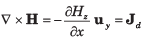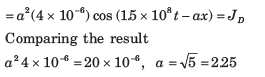QUESTION: 13

Let  H = 2cos(1010 - βx) uz A/m, μ = 3 x 10-5 H/m, ε = 1.2 x 10-10 F/m and σ = 0 everywhere.

Que: The electric flux density D is

Solution: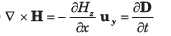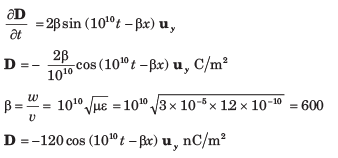QUESTION: 14

Let  H = 2cos(1010 - βx) uz A/m, μ = 3 x 10-5 H/m, ε = 1.2 x 10-10 F/m and σ = 0 everywhere.

Que: The magnetic flux density B is

Solution: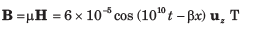QUESTION: 15

A material has  σ = 0 and εr = 1. The magnetic field intensity is H = 4cos ( 106t  - 0.01z)μy A/m.

Que: The electric field intensity E is

Solution: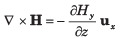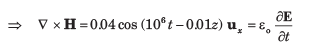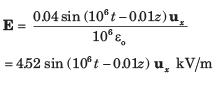QUESTION: 16

A material has  σ = 0 and εr = 1. The magnetic field intensity is H = 4cos ( 106t  - 0.01z)μy A/m.

Que: The value of  μr is

Solution: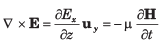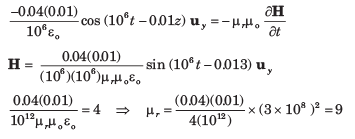QUESTION: 17

The surface ρ = 3 and 10 mm, and z = 0 and 25 cm are perfect conductors. The region enclosed by these surface has μ = 25 x 10-6  H/m, ε = 4 x 10-11 F/m and σ = 0. If H = 2/ρ cos8πz cosωt uø A/m,  then the value of ω is

Solution: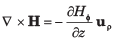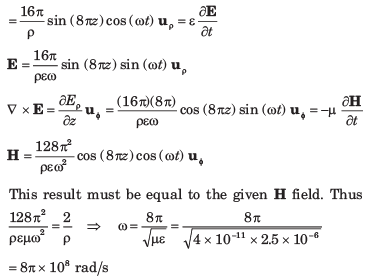QUESTION: 18

For distilled water μ = μo , ε = 81εo and  σ = 2 x 10-3 S/m, the ratio of conduction current density to displacement current density at 1 GHz is

Solution: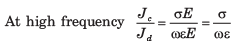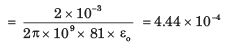QUESTION: 19

A conductor with cross-sectional area of 10 cmcarrier a conductor current 2sin (109 t) mA. If σ = 2.5 x 106 S/m and εr 4.6 the magnitude of the displacement current density is

Solution: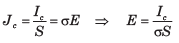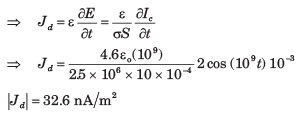QUESTION: 20

In a certain region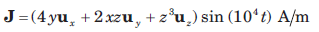If volume charge density ρv in z = 0 plane is zero, then ρv is

Solution: